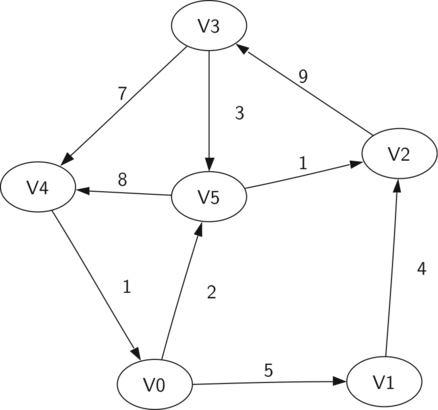# 8.2. Vocabulary and Definitions¶

Now that we have looked at some examples of graphs, we will more formally define a graph and its components. We already know some of these terms from our discussion of trees.

Vertex

A vertex (also called a “node”) is a fundamental part of a graph. It can have a name, which we will call the “key.” A vertex may also have additional information. We will call this additional information the “payload.”

Edge

An edge (also called an “arc”) is another fundamental part of a graph. An edge connects two vertices to show that there is a relationship between them. Edges may be one-way or two-way. If the edges in a graph are all one-way, we say that the graph is a directed graph, or a digraph. The class prerequisites graph shown above is clearly a digraph since you must take some classes before others.

Weight

Edges may be weighted to show that there is a cost to go from one vertex to another. For example in a graph of roads that connect one city to another, the weight on the edge might represent the distance between the two cities.

With those definitions in hand we can formally define a graph. A graph can be represented by $$G$$ where $$G =(V,E)$$. For the graph $$G$$, $$V$$ is a set of vertices and $$E$$ is a set of edges. Each edge is a tuple $$(v,w)$$ where $$w,v \in V$$. We can add a third component to the edge tuple to represent a weight. A subgraph $$s$$ is a set of edges $$e$$ and vertices $$v$$ such that $$e \subset E$$ and $$v \subset V$$.

Figure  2 shows another example of a simple weighted digraph. Formally we can represent this graph as the set of six vertices:

$V = \left\{ V0,V1,V2,V3,V4,V5 \right\}$

and the set of nine edges:

$\begin{split}E = \left\{ \begin{array}{l}(v0,v1,5), (v1,v2,4), (v2,v3,9), (v3,v4,7), (v4,v0,1), \\ (v0,v5,2),(v5,v4,8),(v3,v5,3),(v5,v2,1) \end{array} \right\}\end{split}$Figure 2: A Simple Example of a Directed Graph¶

The example graph in Figure 2 helps illustrate two other key graph terms:

Path

A path in a graph is a sequence of vertices that are connected by edges. Formally we would define a path as $$w_1, w_2, ..., w_n$$ such that $$(w_i, w_{i+1}) \in E$$ for all $$1 \le i \le n-1$$. The unweighted path length is the number of edges in the path, specifically $$n-1$$. The weighted path length is the sum of the weights of all the edges in the path. For example in Figure 2 the path from $$V3$$ to $$V1$$ is the sequence of vertices $$(V3,V4,V0,V1)$$. The edges are $$\left\{(v3,v4,7),(v4,v0,1),(v0,v1,5) \right\}$$.

Cycle

A cycle in a directed graph is a path that starts and ends at the same vertex. For example, in Figure 2 the path $$(V5,V2,V3,V5)$$ is a cycle. A graph with no cycles is called an acyclic graph. A directed graph with no cycles is called a directed acyclic graph or a DAG. We will see that we can solve several important problems if the problem can be represented as a DAG.

You have attempted of activities on this page